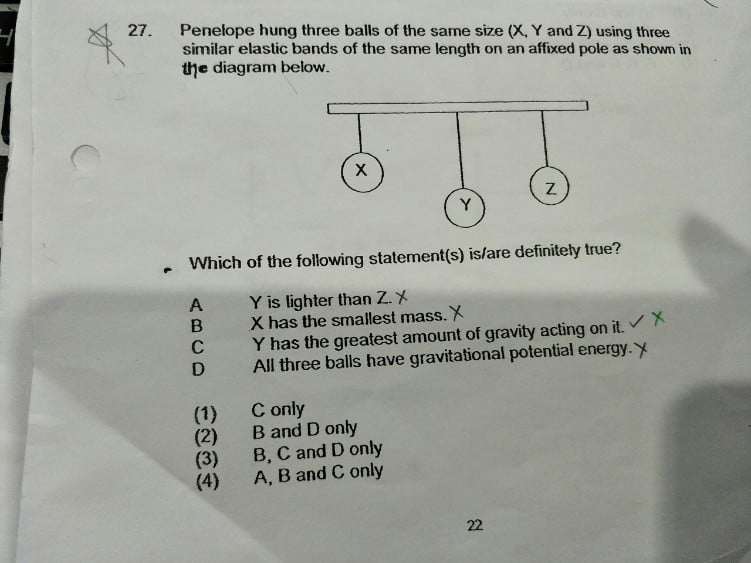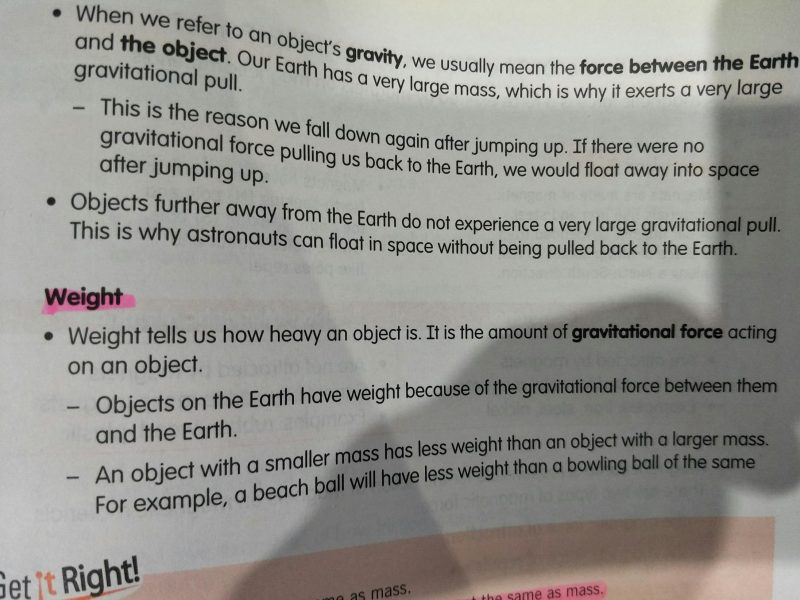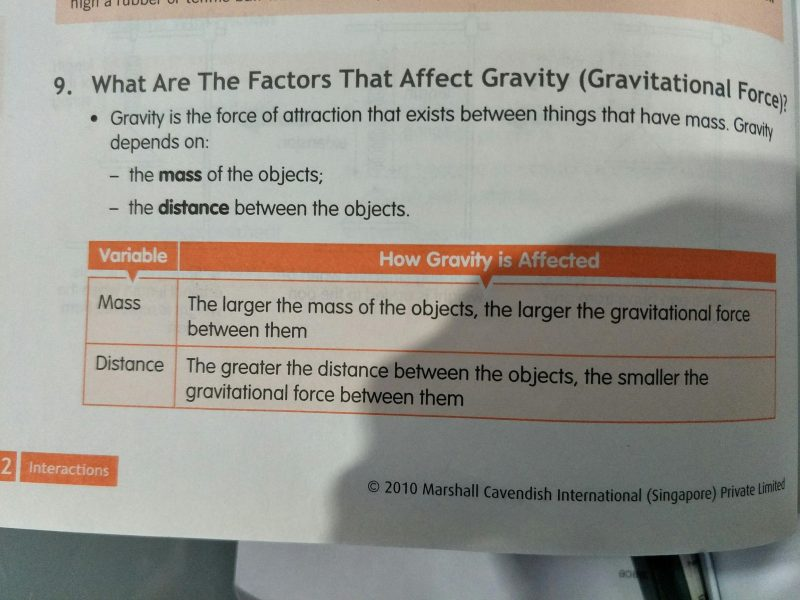QuestionHi,

Should answer be (2) or (3) ?

Is (c) correct ? does pulling the elastic band the most mean that it has the highest mass. As such, it has the greatest amount of gravity acting on it since it depends on mass and distance between objects ?

Thanks for the replies. To a certain extent, i ‘understand’ that gravity acting on all objects on earth is the same. But most books refer gravity to the force of attraction between 2 objects and it depends on the mass and the distance.

So wouldn’t then the gravity on Y is the largest since it has largest mass ?1 Reply 0 Likes

In my opinion, gravity in itself is not a form of energy but gravity creates potential energy. When an object is dropped from higher ground, it is very much like the stored potential energy being released from a loaded mechanical spring converting the stored energy to kinetic energy. Gravity acting on the balls are the same but the gravitational potential energy is different due to different masses.

Hope this clarifies.

0 Replies 0 Likes

Hi,

I think the answer should be 2.

The gravity is the same to all objects in earth. The difference is the air resistance.

0 Replies 0 Likes